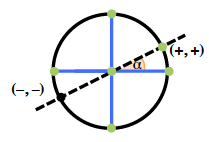### Home > PC3 > Chapter Ch8 > Lesson 8.2.1 > Problem8-68

8-68.

Consider the graphs of $y = \sin\left(x + π\right)$ and $y = -\sin\left(x\right)$.

1. Sketch both graphs on separate axes. What do you notice?

The first curve is shifted left $π$ units.
The second curve is reflected vertically.

2. Sketch the graph of $y = \cos\left(x + π\right)$. What is it equivalent to?

Look at the equations of the curves and the hint in part (a).

3. Use the unit circle to explain why adding $π$ to the angle $x$ reverses the sign of the $x$- and $y$-coordinates.